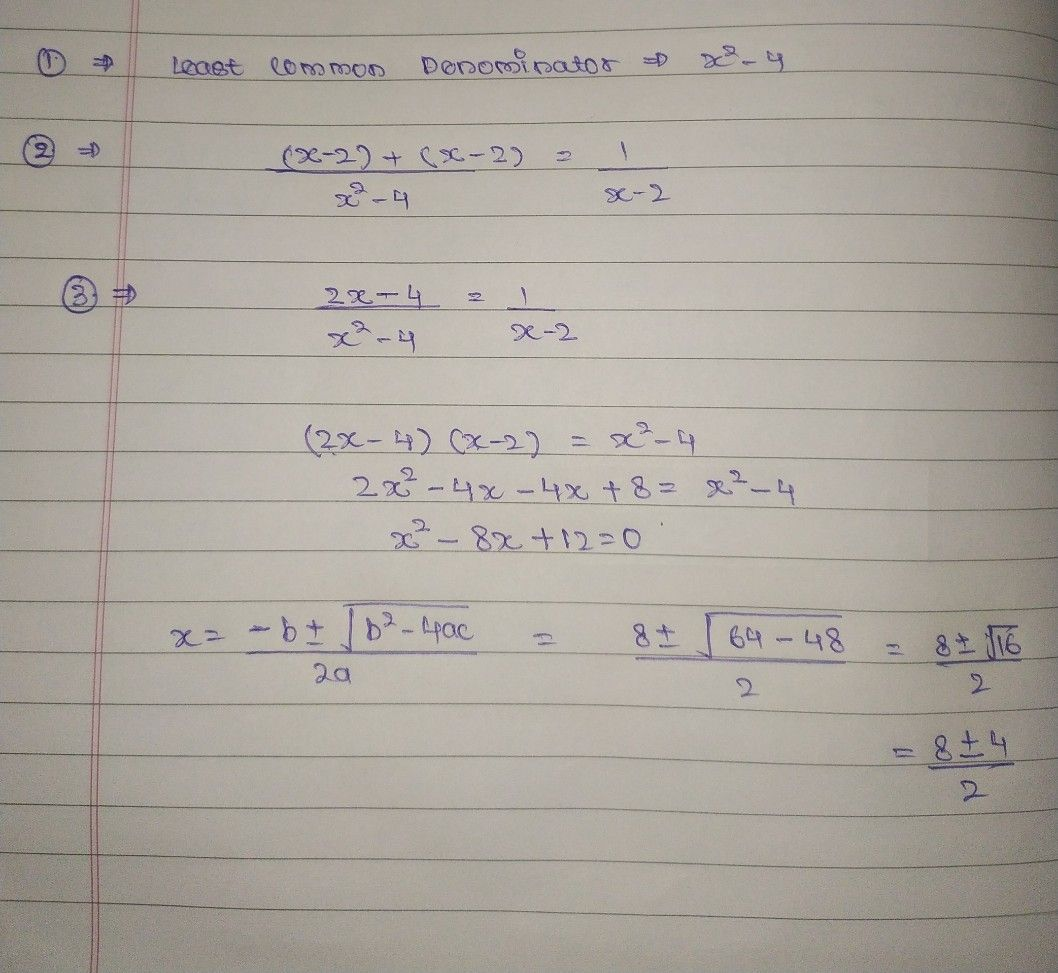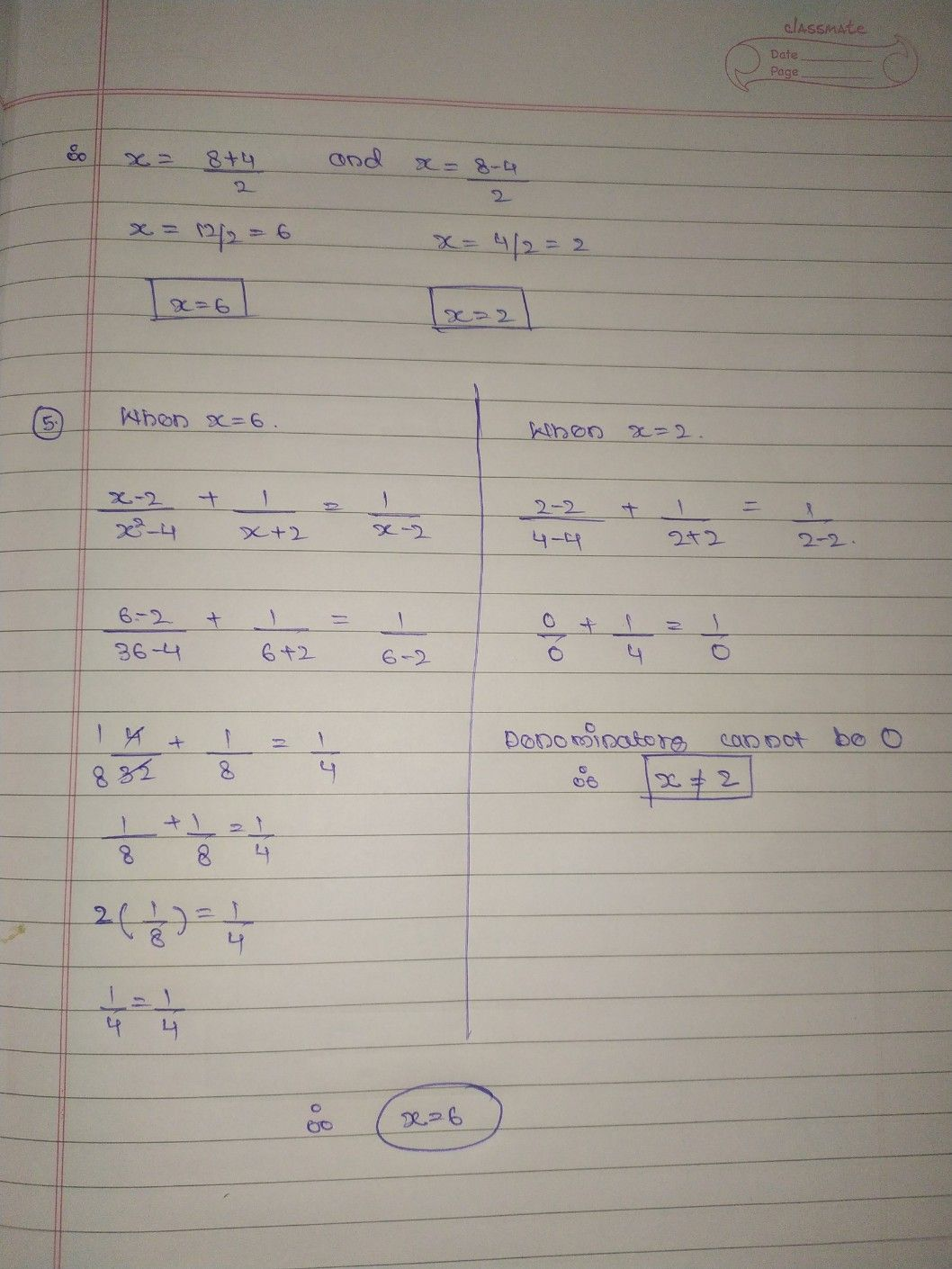Symbol
Problemthe following rational below. $1$ $\dfrac {x-2} {x^{2-4}}+\dfrac {1} {x+2}=\dfrac {1} {x-2}$ Rational Equation $1.$ Find the Least Common Denominator $LCD\right)$ $2$ Multiply both sides of the equation by its the LCD. $3$ Apply the Distributive Property and then simplify. $4$ Find all the possible values of x. $x=6$ $5.$ Check each value by substituting into the original equation and reject any extraneous $oot/s$ $S$
10th-13th grade
Algebra
Search count: 110
SolutionQanda teacher - Shewtaplease ask if any doubts